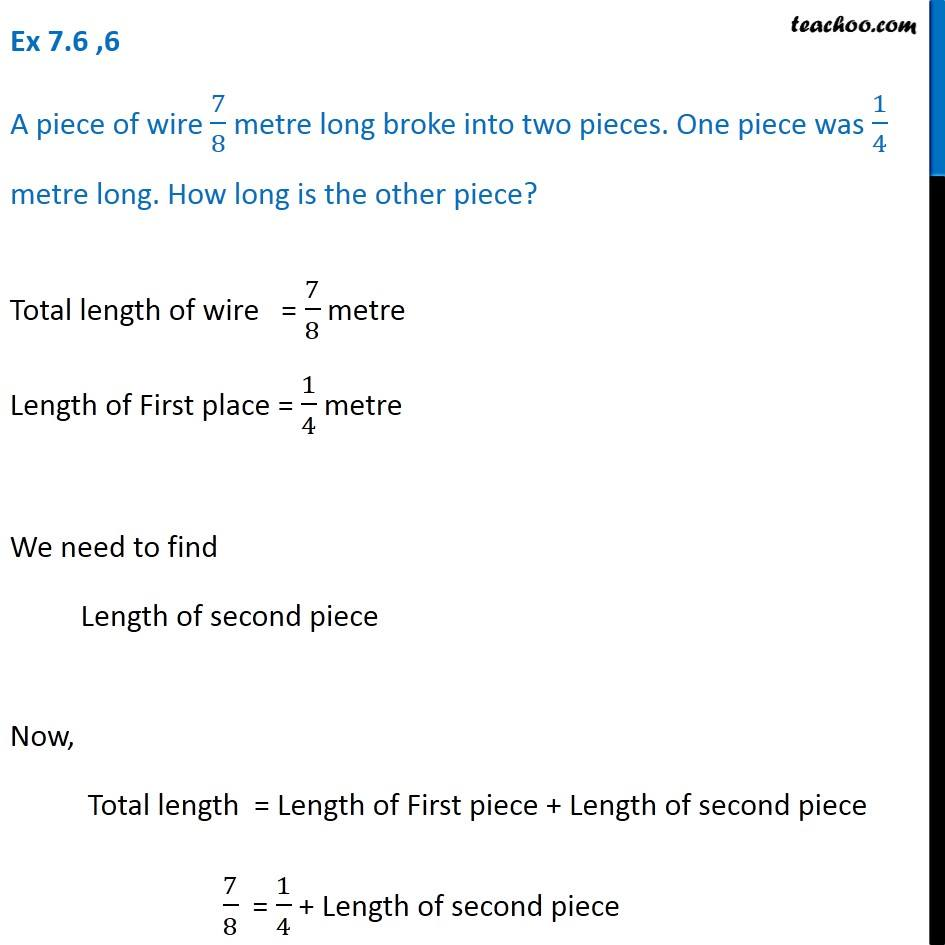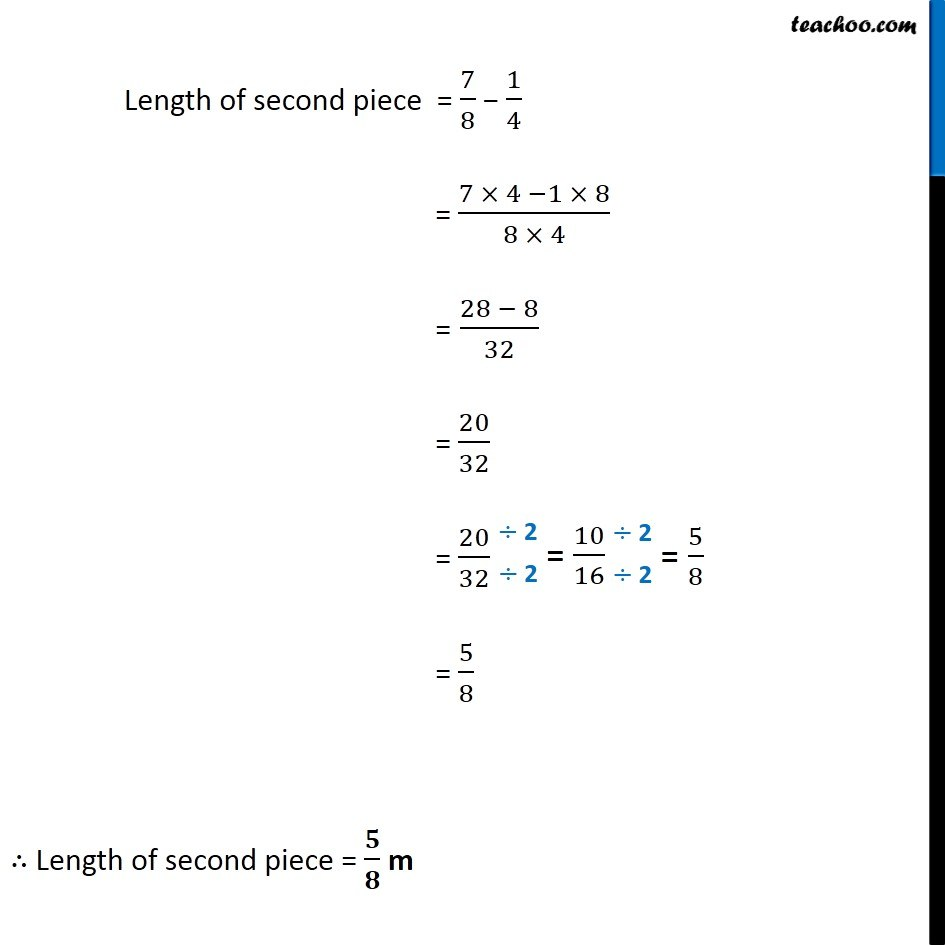1. Chapter 7 Class 6 Fractions
2. Concept wise
3. Statement Questions - Addition and Subtraction of Fractions

Transcript

Ex 7.6 ,6 A piece of wire 7/8 metre long broke into two pieces. One piece was 1/4 metre long. How long is the other piece? Total length of wire = 7/8 metre Length of First place = 1/4 metre We need to find Length of second piece Now, Total length = Length of First piece + Length of second piece 7/8 = 1/4 + Length of second piece Length of second piece = 7/8 1/4 = (7 4 1 8)/(8 4) = (28 8)/32 = 20/32 = 20/32 = 5/8 Length of second piece = / m

Statement Questions - Addition and Subtraction of Fractions